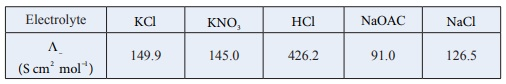# UNIT 9: Electro Chemistry - Online Test

Q1. The number of electrons that have a total charge of 9650 coulombs is
Explaination / Solution:

1F = 96500 C = 1 mole of e = 6.023 ×1023 e

9650 C = [ 6.22 ×1023 / 96500 ] × 9650 = 6.022×1022

= 6.022×1022

Q2.

Consider the following half cell reactions:

Mn2+ + 2e Mn   Eº = -1.18V

Mn2+ Mn3+ + e-   Eº = -1.51V

The E for the reaction 3Mn2+ Mn+2Mn3+ , and the possibility of the forward reaction are respectively.

Explaination / Solution:

Mn2+ + 2e Mn (Eºred) = -1.18V

2[Mn2+ Mn3+ + e- ](Eºox) = −1.51V

3Mn2+ Mn + 2Mn3+        Eºcell?

Eºcell = ( Eºox )+ ( Eºred )

= −1.511.18 and non spontaneous

= −2.69V

Since Eo is –ve ∆G is +ve and the given forward cell reaction is non – spontaneous.

Q3.

The button cell used in watches function as follows

Zn (s) + Ag2 O (s) + H2 O (l) 2 Ag (s) + Zn2+ (aq) + 2OH (aq) the half cell potentials are Ag2 O (s) + H2O (l) + 2e 2Ag (s) + 2 OH (aq) E = 0.34V The cell potential will be
Explaination / Solution:

Anodic oxidation: (Reverse the given reaction)

( Eoox ) = 0.76V cathodic reduction

Ecell = ( Eoox )+ ( Eredo )

= 0.76 + 0.34 = 1.1V

= 1.1V

Q4. The molar conductivity of a 0.5 mol dm-3 solution of AgNO3 with electrolytic conductivity of 5.76 ×103 S cm1 at 298 K is
Explaination / Solution:

Λ = κ/M ×103 mol1 m3

= [( 5.76 × 103 S cm1 ×103 ) / (0. 5)]  mol1m3

= [(5.76 × 103 × 103 ×106 ) / (0.5)] S cm1mol1 cm3 .

= 11.52 S cm2 mol1

Q5.Calculate ΛºHOAC using appropriate molar conductances of the electrolytes listed above at infinite dilution in water at 25oC .
Explaination / Solution:

( Λº )HoAC = ( Λº)HCl + ( Λº)NaOAC - ( Λº)NaCl

= (426.2 + 91) (126.5)

= 390.7

Q6. Faradays constant is defined as
Explaination / Solution:

1F = 96500 C = charge of 1 mole of e- =

charge of 6.022 ×1023 e

Q7. How many faradays of electricity are required for the following reaction to occur MnO-4 Mn2+
Explaination / Solution:

7MnO-4 + 5e Mn2+ + 4H2O

5 moles of electrons i.e., 5F charge is required.

Q8. A current strength of 3.86 A was passed through molten Calcium oxide for 41minutes and 40 seconds. The mass of Calcium in grams deposited at the cathode is (atomic mass of Ca is 40g / mol and 1F = 96500C).
Explaination / Solution:

m=ZIt

(41min 40sec = 2500 seconds)

Z = m/ (n × 96500) = 40 / (2 × 96500)

m = Zit

= ( 40 × 3.86 × 2500 ) / (2 × 96500)

= 2g

Q9. During electrolysis of molten sodium chloride, the time required to produce 0.1mole of chlorine gas using a current of 3A is
Explaination / Solution:

m=ZIt

(mass of 1 mole of Cl2 gas = 71)

t = m/ ZI  (mass of 0.1mole of Cl2 gas = 7.1 g mol1 )

= 7.1 / [71/(2 ×96500) × 3 ]

(2 Cl- → Cl2 +2e- )

= (2 × 96500 × 7.1) / (71× 3)

= 6433.33sec

= 107.2 min

Q10. The number of electrons delivered at the cathode during electrolysis by a current of 1A in 60 seconds is (charge of electron = 1.6 ×1019 C )
Explaination / Solution:

Q =It

= 1A×60S

96500 C charge ≡ 6.022 ×1023 electrons

60 C charge ≡ [ 6.022 ×1023 /  96500  ] × 60

= 3.744 ×1020 electrons